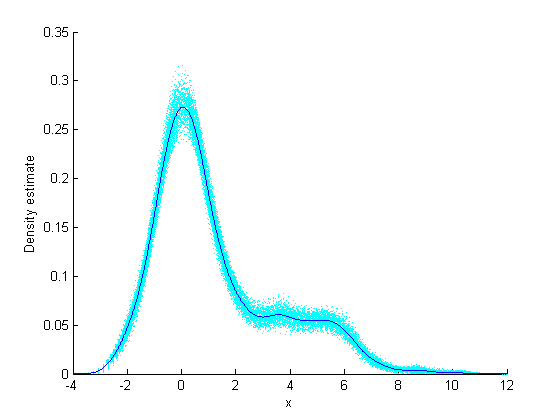## Implement Bootstrap Using Parallel Computing

### Bootstrap in Serial and Parallel

Here is an example timing a bootstrap in parallel versus in serial. The example generates data from a mixture of two Gaussians, constructs a nonparametric estimate of the resulting data, and uses a bootstrap to get a sense of the sampling variability.

1. Generate the data:

```% Generate a random sample of size 1000, % from a mixture of two Gaussian distributions x = [randn(700,1); 4 + 2*randn(300,1)];```
2. Construct a nonparametric estimate of the density from the data:

```latt = -4:0.01:12; myfun = @(X) ksdensity(X,latt); pdfestimate = myfun(x);```
3. Bootstrap the estimate to get a sense of its sampling variability. Run the bootstrap in serial for timing comparison.

```tic;B = bootstrp(200,myfun,x);toc Elapsed time is 10.878654 seconds.```
4. Run the bootstrap in parallel for timing comparison:

```mypool = parpool() Starting parpool using the 'local' profile ... connected to 2 workers. mypool = Pool with properties: AttachedFiles: {0x1 cell} NumWorkers: 2 IdleTimeout: 30 Cluster: [1x1 parallel.cluster.Local] RequestQueue: [1x1 parallel.RequestQueue] SpmdEnabled: 1 ```
```opt = statset('UseParallel',true); tic;B = bootstrp(200,myfun,x,'Options',opt);toc Elapsed time is 6.304077 seconds.```

Computing in parallel is nearly twice as fast as computing in serial for this example.

Overlay the `ksdensity` density estimate with the 200 bootstrapped estimates obtained in the parallel bootstrap. You can get a sense of how to assess the accuracy of the density estimate from this plot.

```hold on for i=1:size(B,1), plot(latt,B(i,:),'c:') end plot(latt,pdfestimate); xlabel('x');ylabel('Density estimate')```### Reproducible Parallel Bootstrap

To run the example in parallel in a reproducible fashion, set the options appropriately (see Running Reproducible Parallel Computations). First set up the problem and parallel environment as in Bootstrap in Serial and Parallel. Then set the options to use substreams along with a stream that supports substreams.

```s = RandStream('mlfg6331_64'); % has substreams opts = statset('UseParallel',true,... 'Streams',s,'UseSubstreams',true); B2 = bootstrp(200,myfun,x,'Options',opts);```

To rerun the bootstrap and get the same result:

```reset(s) % set the stream to initial state B3 = bootstrp(200,myfun,x,'Options',opts); isequal(B2,B3) % check if same results ans = 1```
﻿